# Magic Squares: Logical Thinking

I’m looking at various common puzzles, and ways to think about them logically. Today, we’ll examine basic magic squares: not the standardized methods you can look up, but how a kid can work them out when seeing them for the first time. Often they are not even told they have a name, so there is nothing to look up! But there is some great thinking to be done.

## Reflective trial and error

I’ll start with a relatively simple version of the problem, from 2006:

Number Puzzle with Digits 1-9 in a 3 by 3 Grid

In a 3 cell by 3 cell grid, use the digits 1 through 9 to fill in the cells so each horizontal, vertical, and diagonal row of three has a sum of 15.  No digit may be used more than once.

I've tried multiple ways with no solution!  Frustration!  This puzzle is my 4th grader's POW (Parent Over Worked).  I have no idea why my son's math teacher would send something like this home other than to have the parents do it.

Here the class is given the numbers to use and the total for each row (which is a significant help: We’ll later see it being left for the student to work out). We have to fill this in: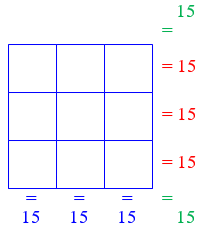If you don’t know anything about the puzzle, it can seem like a lot of busy work at best, and frustrating trial and error at worst. And, yes, a lot of students probably come away frustrated. But, in fact, trial and error is a valuable skill, and a parent’s primary role may be to encourage patience.

This is a standard (and ancient) puzzle called a Magic Square.  I'm not sure what the point is in assigning it, myself: many people know about it and can either look it up or build it using some fairly simple rules; those who don't just have to use lots of trial and error.  I don't know how one would work it out using any specifically "mathematical" ideas, apart from those very specific techniques for building magic squares.  I would hope, since it is so unfair as a test of any particular skill, that its purpose is simply FUN.  I'm sure it's not meant to frustrate kids or make their parents do a lot of work.  Enjoyment of puzzles is one of the foundations of later math skill!

So how would I approach it as a fourth-grader?

I'll pretend I've never heard of the puzzle, and try talking through what I might do to solve it by trial and error.  Maybe that will give you a start at how to guide your child to work on it, and perhaps even enjoy it.

I'll just draw the grid as
_ _ _
_ _ _
_ _ _

Let's suppose we just start by trying the 1 in the left corner:

1 _ _
_ _ _
_ _ _

We have to be able to get 15 in the horizontal row, the vertical row, AND the diagonal containing the 1!  So we need to make 14 using two numbers, in three different ways.  Let's see ... that would be 9+5, 8+6, and 7+7 -- oops!  We can't do it, since the numbers have to be different.  So 1 can't be in a corner.  We've learned something important!

We need to fill in three pairs that each add to 14, and we can’t do it: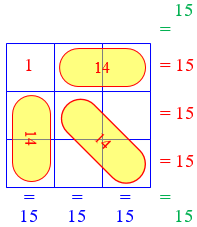An important rule for solving unfamiliar puzzles is to just try something! Whatever you do, if you pay attention, there will be things to learn, even if they are only what not to do. (Some of what we’re learning here will be used more deliberately in a more advanced answer later.)

How about putting 2 in the corner; then we need three pairs of numbers that each add up to 13 so that the total in each direction is 15:

2 _ _
_ _ _
_ _ _

We can use 9+4, 8+5, and 7+6.  That looks better.  But where shall we put them?  Let's just try one possibility, and see what goes wrong that we could fix:

2 9 4
7 8 _
6 _ 5

Again, I’ve just tried something, to see what I can learn: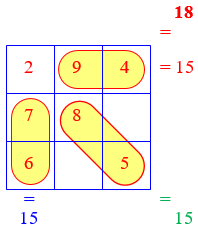Again, we don’t have to know what we’re doing; we’re just feeling our way around to learn about the nature of the problem. I arbitrarily put each pair in some direction, and in decreasing order going away from the 2. (My trained instincts actually tell me that might be the worst thing to do, but I’m deliberately ignoring that, in order to demonstrate how to learn.)

I’ve put in a row, a column, and a diagonal; what other three-in-a-row have I made?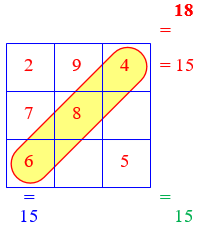First, I see we've made a diagonal; does that add up to 15?  6+8+4 = 18, not 15.  So maybe the first thing we have to do is to pick one number from each pair to go on the diagonal. We need to take away 3 from the sum; we could do that by just swapping the 8 with the 5.  And in fact, that's the ONLY way without moving the pairs themselves to new places, since swapping in the other pairs will always INCREASE the sum.  So now we have

2 9 4
7 5 _
6 _ 8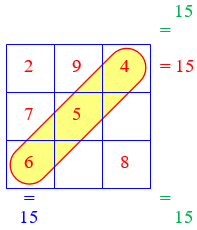Rather than arbitrarily place the pairs, I needed to intentionally place numbers on the diagonal. But in fact, I could use my naive first placement as a basis for a modification. Nothing I’ve done has been wasted, because I’m being observant.

Four of the six sums are correct! I've taken you almost all the way to a solution, though maybe not to the only one, as far as we can tell, since there are a lot of rearrangements I didn't try (putting a different pair than 5,8 on the diagonal, for example, or putting 3 in the corner). I'll let you finish, and then try guiding your child to find a solution.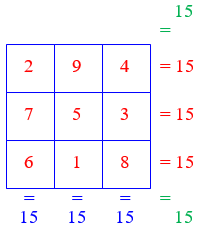Note that because we are just using trial and error, we don’t know whether there are other solutions; but at this level, we’ve had a great success in finding a solution at all!

The sort of thinking I've just demonstrated is basic mathematical reasoning--we go through possibilities in an orderly way, looking at  what happens and making adjustments.  I don't know whether this sort of thing is taught in 4th grade (or, perhaps, can really be taught at all); but I think the main benefit in assigning puzzles like this is the opportunity for students to discuss their reasoning and develop the skill of talking about how they think.  This "reflective thought" is an important part of good learning, and I hope your child gets a chance to do it, either with you or in class or with fellow students at other times.

It was fun talking about my thinking here (also called metacognition – being aware of how you are thinking, which is a necessary part of developing greater skills), and I hope it was a good model for both parent and child. The father responded,

Thanks for the help and the quick response.  Sometimes things that seem simple can be very frustrating!  With your very detailed instructions it made it understandable.

## Methodical reasoning

An older student can use a more orderly method; in effect, we will just be front-loading much the same kind of thinking we did in the trial and error approach. Here is a question from 2005:

Solving a 3 x 3 Magic Square

Place the numbers 1-9 in a 3 by 3 grid, one number per box, so that the vertical, horizontal, and diagonal sums are all the same.

I have tried and tried to figure this out, but I can't seem to get it.  I know that small numbers should be on the end because that is what our teacher said, and I'm pretty sure the middle number is 9 but I just haven't been able to find the answer.

I’m not sure what the hint about small numbers means. The middle-number guess is wrong (as we’ll specifically see in our last example below).

Doctor Wilko took this one:

You could certainly use a "trial and error" approach and get a correct solution to the 3x3 magic square, but it sounds like you've tried that without luck, so let's try a more methodical approach.

One way a 3x3 magic square can be constructed is by using a little simple arithmetic and algebraic reasoning.

The first issue we face is that in this version of the problem, we haven’t been told what the sum of each row (the “magic number”) has to be. Can we figure it out? This is a classic trick:

One could reason that the numbers 1-9 add up to 45, and since all these numbers are contained in three ROWS (exclusively), the sum of each ROW must be 15.  I want to make sure you understand this before proceeding, so look at the little algebraic argument below that "proves" that each row should sum to 15:

This quick explanation in words is enough for some people, but an explicit demonstration will help others:

Since we don't know the order of the numbers 1-9 that correctly fill out the 3x3 magic square, substitute the variables a-i into the square.

+---+---+---+
| a | b | c |
+---+---+---+
| d | e | f |
+---+---+---+
| g | h | i |
+---+---+---+

We know that

a + b + c + d + e + f + g + h + i = 45

grouping the letters by rows we get

(a + b + c) + (d + e + f) + (g + h + i) = 45

But since the sum of each row should be equal (let's say the sum equals X), the rows can be rewritten as

(a + b + c) = X
(d + e + f) = X
(g + h + i) = X

and the equation above can then be rewritten as

X + X + X = 45, or

3*X = 45, so

X = 15

This shows that the three rows sum to 15.

It then follows from the definition of a magic square that all the columns and diagonals will also sum to 15.

In some puzzles (as we’ll see next time), a key number like this is not determined by the problem; but in magic squares, it is. That’s an important first step.

Now we have to think about how to arrange the numbers to get that sum. Rather than discover the issues that will arise by trial, Doctor Wilko considers them ahead of time:

OK, now we know that each row, column, and diagonal will sum to 15, so the challenge is to figure out HOW to arrange the numbers to make that happen.

This may be done by a brute-force method as follows.  Basically, through trial and error, I found all the ways that I could take three numbers from 1-9 and get a sum of 15:

1 + 5 + 9 = 15
1 + 6 + 8 = 15
2 + 4 + 9 = 15
2 + 5 + 8 = 15
2 + 6 + 7 = 15
3 + 4 + 8 = 15
3 + 5 + 7 = 15
4 + 5 + 6 = 15

Again, double check me here!  Convince yourself that these are the only ways you can get a sum of 15 from three numbers chosen from 1-9.

I can see that in making this list, he has actually used, not a random trial and error method, but an orderly search. He kept the numbers in increasing order in each sum, and started with the first number being 1, then the second number being the smallest possible value. The result is a list much like a list of numbers in increasing order (158, 168, 249, …) This way, we can be sure that nothing was missed. This is closely related to the way I started with 1 in the corner and listed pairs that add to 14, then tried 2. (Do you see my list of pairs that sum to 14 hidden in there?)

For what he does next, I find it helpful to write the sums as follows, with each number assigned a column; this also shows a pattern in the numbers that helped in making the list:

  1             + 5             + 9 = 15
1                 + 6     + 8     = 15
2     + 4                 + 9 = 15
2         + 5         + 8     = 15
2             + 6 + 7         = 15
3 + 4             + 8     = 15
3     + 5     + 7         = 15
4 + 5 + 6             = 15

Here we can see where each individual number fits into the list. By the way, did you notice that there are 8 sums here, and we’ll need each of them, since there are 3 rows, 3 columns, and 2 diagonals, for a total of 8 sets of three to add? That suggests how “magical” a magic square really is!

Now, from the equations above, note the following connections to the 3x3 magic square:

1, 3, 7, and 9 are each in TWO equations that sum to 15.  The middle cells of each outside row/column are each in TWO equations (One row, one column).

+---+---+---+
| - | x | - |
+---+---+---+
|   | | |   |
+---+---+---+
|   | | |   |
+---+---+---+

(I'm willing to bet that 1, 3, 5, and 7 will each fill one of these middle cells on the outside rows/columns!)

We’re exhaustively examining each possible type of cell, and comparing them to our exhaustive list of sums.

2, 4, 6, and 8 are each in THREE equations that sum to 15.  The corner cells are each in THREE equations (One row, one column, and one diagonal).

+---+---+---+
| x | - | - |
+---+---+---+
| | | \ |   |
+---+---+---+
| | |   | \ |
+---+---+---+

(From this, I'd be willing to bet that 2, 4, 6, and 8 are going to fill the corners of the magic square!)

5 is in FOUR equations that sum to 15.  The middle cell is in FOUR equations (one row, one column, and two diagonals).

+---+---+---+
| \ | | | / |
+---+---+---+
| - | x | - |
+---+---+---+
| / | | | \ |
+---+---+---+

(From this it seems likely that 5 should go in the middle cell of the 3x3 magic square!)

Now we’re all set to start actually build a magic square with confidence (having bypassed all the “errors”, so our “trials” will work):

Using the above observations, let's fill out the square!

Looking at a blank 3x3 magic square, one can see that the middle cell should be in four equations (one row, one column, and two diagonals), and looking at the equations, one can see that 5 is the only number in four of the eight equations.  Therefore, 5 can be put into the middle cell.

+---+---+---+
|   |   |   |
+---+---+---+
|   | 5 |   |
+---+---+---+
|   |   |   |
+---+---+---+

Now there are four corners which when filled, are each used in three equations.  By putting any of the four numbers (2,6,4,8) in the upper-left corner (2 in this example), only one number can go in the bottom-right corner (8 in this example) to make the sum of that diagonal be 15.

+---+---+---+
| 2 |   |   |
+---+---+---+
|   | 5 |   |
+---+---+---+
|   |   | 8 |
+---+---+---+

Now, either of the other numbers (4 or 6 in this example) can go in the bottom left corner (6 in this example), leaving only one choice for the top right corner (4 in this example).

+---+---+---+
| 2 |   | 4 |
+---+---+---+
|   | 5 |   |
+---+---+---+
| 6 |   | 8 |
+---+---+---+

Last, the remaining four numbers (1, 3, 7, 9) can be placed in only one way to make the sums be 15.

+---+---+---+
| 2 | 9 | 4 |
+---+---+---+
| 7 | 5 | 3 |
+---+---+---+
| 6 | 1 | 8 |
+---+---+---+

This is one correct solution to the 3x3 magic square.

There are seven other solutions.  A 3x3 magic square contains eight possible solutions total; four rotations and four reflections.

We had only two points where we made a choice here: We picked one of 4 possibilities for the upper left corner, and then one of 2 possibilities for the upper right. That makes a total of 8 ways we could have done it; and each of these can be obtained by rotating what we did, or reflecting it. So in a sense all 8 solutions are really the same solution placed differently. By chance, we got the very same solution as before.

## What can go in the middle?

Let’s look at one more question of this sort, this one from 2001:

Magic Square Variations

A magic square consists of numbers arranged in a square so that all rows, columns, and usually the two diagonals will add up to the same sum. Try to create a magic square by arranging the first nine counting numbers in the nine square cells.

There is only one possible way. Can you please help me?

This is the usual 3×3 magic square question. As we’ve seen, it’s not quite right to say there is only one way to do it. Doctor Jeremiah answered, first finding the “magic sum”:

There are actually 8 different ways, but they are all rotations and mirror images of the same one.

First consider that if you have 3 cells wide by 3 cells high, you will have to put the numbers 1 through 9 in these nine cells. The sum of the diagonals, rows, and columns will be the same.

That means that the sum of all three columns must be the same as the sum of all nine numbers because the nine numbers fit into the three columns.  (Let's call the sum of a column, row, or diagonal S):

sum of 3 columns =      sum of all nine numbers

3S         = 1 + 2 + 3 + 4 + 5 + 6 + 7 + 8 + 9

3S         = 1 + 2 + 3 + 4 + 5 + 6 + 7 + 8 + 9
3S         = 45
3S / 3     = 45 / 3
S         = 15

So the "magic sum" is 15.

Next, rather than list all possible sums, he focused on the middle cell, using inequalities:

Let's think a bit about what number must be in the middle. It's an important number because it is used in every sum (all the rows, columns and diagonals).

If that middle cell holds 6, then what? In which cell can you put the 9? You can't put it anywhere, because 6+9 = 15 with only two numbers, and we need to make _three_ numbers add to 15. So 6 and above cannot be in the middle cell.

If that middle cell holds 4, then what? In which cell can you put the 1? You can't put it anywhere, because 4+1 = 5, and to make 15 you need to put 10 into a cell, but 10 isn't a choice because it's not one of the 9 counting numbers. So 4 and below cannot be in the middle cell.

That leaves 5. With 5 in the middle cell the solution is easy (especially if you know the magic sum is 15).

He left the “patient” with just these hints. A good next step might be to observe that opposite cells will all have to sum to 10.

Another question we’ve answered dealt with a modified version of the question: a 4×4 square with a special set of numbers, and no diagonals to worry about:

Almost a Magic Square

Most of the questions in the Ask Dr. Math archive about magic squares tell about routine methods to be learned for making magic squares of various sizes, without talking about how those algorithms would have been invented. I’ll eventually put those into a post, but here are a couple of them if you are interested:

Finding Magic Squares (odd sizes)

How to Create a 4 x 4 Magic Square

Even-Numbered Magic Squares

Next time, I’ll look at some “magic squares” (and triangles and pentagons) that use only the edges of a figure, not a solid square.

This site uses Akismet to reduce spam. Learn how your comment data is processed.